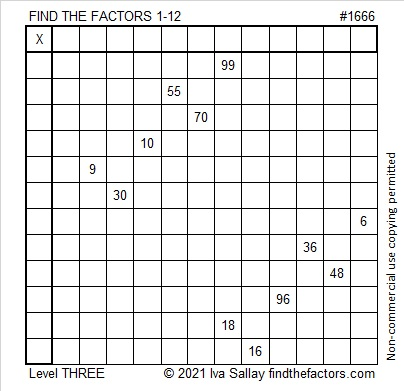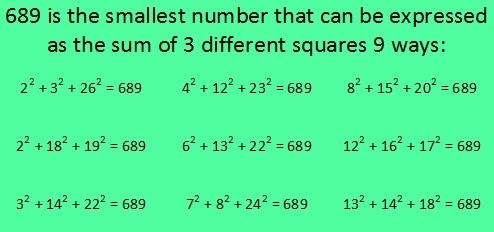# 1666 Demystifying a Tricky Puzzle

### Today’s Puzzle:

This puzzle isn’t as tricky as it could be simply because I arranged its clues into a level 3 puzzle. That means that after you write the factors of 99 and 18 in the appropriate boxes in the first column and top row, you work your way down the puzzle clue by clue in the order they appear. Still, you will have to think about what to do with the 70, but I think you can handle it!### Factors of 1666:

• 1666 is a composite number.
• Prime factorization: 1666 = 2 × 7 × 7 × 17, which can be written 1666 = 2 × 7² × 17.
• 1666 has at least one exponent greater than 1 in its prime factorization so √1666 can be simplified. Taking the factor pair from the factor pair table below with the largest square number factor, we get √1666 = (√49)(√34) = 7√34.
• The exponents in the prime factorization are 1, 2, and 1. Adding one to each exponent and multiplying we get (1 + 1)(2 + 1)(1 + 1) = 2 × 3 × 2 = 12. Therefore 1666 has exactly 12 factors.
• The factors of 1666 are outlined with their factor pair partners in the graphic below.### More About the Number 1666:

1666 is the sum of two squares because ALL of its odd prime factors either leave a remainder of 1 when divided by 4 OR have an even exponent:
1666 = 2 × 7² × 17,
17÷4 = 4 R1, The exponent on 7² is even.
What are the two squares?
35² +  21² = 1666.

1666 is the hypotenuse of a Pythagorean triple :
784-1470-1666 which is (8-15-17) times 98 and
can also be calculated from 35² –  21², 2(35)(21), 35² +  21².

# What Makes 689 Amazing?

689 is an amazing number for several reasons. I decided to make graphics to illustrate many of those ways. (689’s factoring information is at the end of this post.)

689 is the sum of consecutive prime numbers 227, 229, and 233.

Also 689 is the sum of the primes from 83 to 109. Do you know what those 7 prime numbers are?

OEIS.org informs us that 689 is the smallest number that can be expressed as the sum of three different square numbers NINE ways. I decided to figure out what those nine ways are and make this first graphic to share with you:Note: 614 can also be expressed as the sum of 3 squares 9 different ways, but one of those ways is 17² + 17² + 6² = 614, and that duplicates 17² in the same sum.

689 is the same number when it is turned upside down. Numbers with that characteristic are called Strobogrammatic numbers.689 BASE 10 isn’t a palindrome, but 373 BASE 14 is; note that 3(196) + 7(14) + 3(1) = 689

Both of 689’s prime factors have a remainder of 1 when divided by 4, so they are hypotenuses of Pythagorean triples. That fact also means 689 can be expressed as the sum of two square numbers TWO different ways, and it makes 689 the hypotenuse of FOUR Pythagorean triples.  Can you tell by looking at the graphic which two are primitive and which two aren’t?689 is the sum of consecutive numbers three different ways:

• 344 + 345 = 689; that’s 2 consecutive numbers.
• 47 + 48 + 49 + 50 + 51 + 52 + 53 + 54 + 55 + 56 + 57 + 58 + 59 = 689; that’s 13 consecutive numbers.
• 14 + 15 + 16 + 17 + 18 + 19 + 20 + 21 + 22 + 23 + 24 + 25 + 26 + 27 + 28 + 29 + 30 + 31 + 32 + 33 + 34 + 35 + 36 + 37 + 38 + 39 = 689; that’s 26 consecutive numbers.

Now you have a few reasons why 689 is an amazing number. 13 and 53 were part of some of those reasons so it shouldn’t surprise anyone to see 13 and 53 pop up in its factoring information, too:

——————————————————————————

• 689 is a composite number.
• Prime factorization: 689 = 13 x 53
• The exponents in the prime factorization are 1 and 1. Adding one to each and multiplying we get (1 + 1)(1 + 1) = 2 x 2 = 4. Therefore 689 has exactly 4 factors.
• Factors of 689: 1, 13, 53, 689
• Factor pairs: 689 = 1 x 689 or 13 x 53
• 689 has no square factors that allow its square root to be simplified. √689 ≈ 26.248809.